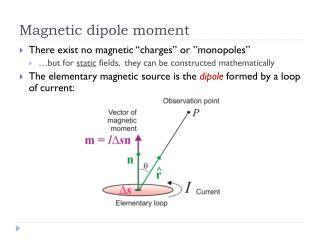DownloadDownload PresentationMagnetic dipole moment

# Magnetic dipole moment

Télécharger la présentation## Magnetic dipole moment

- - - - - - - - - - - - - - - - - - - - - - - - - - - E N D - - - - - - - - - - - - - - - - - - - - - - - - - - -
##### Presentation Transcript

1. Magnetic dipole moment • There exist no magnetic “charges” or ”monopoles” • …but for static fields, they can be constructed mathematically • The elementary magnetic source is the dipole formed by a loop of current:

2. Magnetic Potential • If curl (B) = 0 (no current), field B can be presented as a gradient of a potential V: or: • Biot-Savart law: Cm depends on units

3. Magnetic Potential • Therefore, the change in potential: The first equation here uses: The second equation assumes an infinitesimal loop • The contour integral gives the area of the loop: • Hence the change in potential:

4. Magnetic Potential • Therefore, the magnetic potential equals: The const is usually taken zero, so that • Note two properties different from the monopole potential for gravity: • The potential decays with distance as 1/r2 • It also depends on the direction (zero within the plane orthogonal to m and opposite signs in the directions parallel and opposite to m)

5. Poisson’s relation • The dipole field (~1/r2) equals a derivative of the monopole field (~1/r) with respect to the position of the source: This is simply the spatial derivative in the direction of the magnetic moment, m • Thus the magnetic field of a structure is related to its gravity field (Poisson’s relation):

6. Monopole Magnetic Potential • The dipole field can be presented as produced by two monopolesseparated by an arbitrary (small) distance a: where and is the pole strength